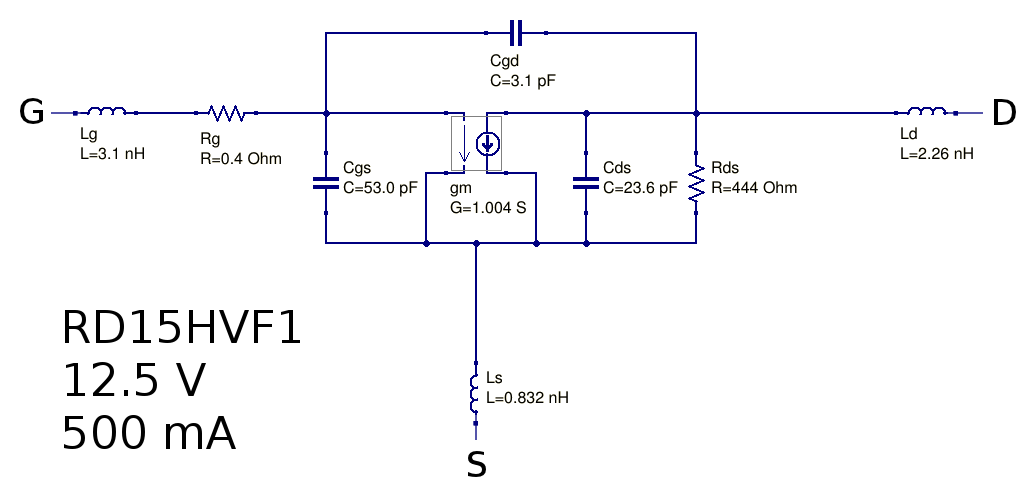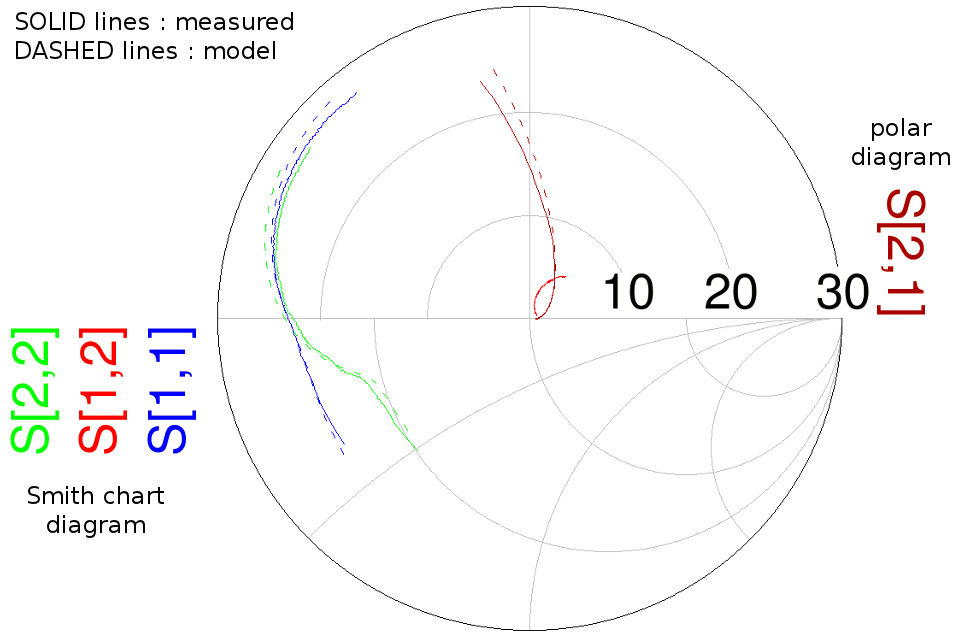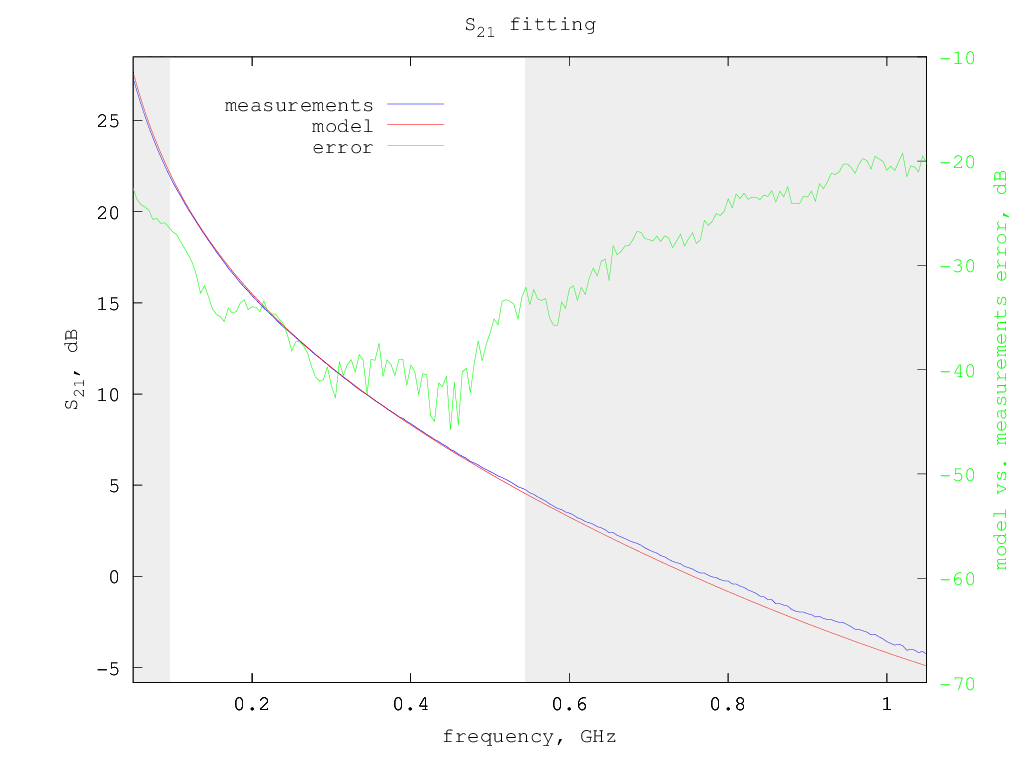# Mitsubishi RD15HVF1 LDMOS model

Under construction...

The Mitsubishi RD15HVF1 is a medium power LDMOS, quite similar to its cousin RD16HHF1 and like this latter often used to build small amplifiers for amateur radio for the HF bands, even if it can be used also into the upper-VHF frequencies.

# Model for Vdd=12.5 V and Idd= 500 mA

Typical S-parameters for this device can be found on the Mitsubishi web site, for a supply voltage of 12.5 V and a drain current of 500 mA.

Using the extraction method previously described, considering only the S-parameters from 50 MHz to 500 MHz, the following small-signal model parameters are determined:

```Rg = 0.4 ohm
Rd = 0 ohm
Rs = 0 ohm
Lg = 3.1 nH
Ld = 2.26 nH
Ls = 0.832 nH
Cgs = 53.0 pF
Cds = 23.6 pF
Cgd = 3.1 pF
gm = 1.004 S
gds = 2.25 mS
```
So the equivalent small-signal circuit for this LDMOS at 500 mA drain current is the following:Smith chart with measured vs. model S-parameters:S-parameters fitting details - fitted region is in white background: